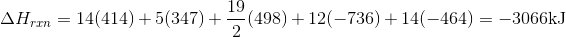# College Chemistry : Standard Enthalpy and Entropy of Reactions

## Example Questions

### Example Question #1 : Solutions, States Of Matter, And Thermochemistry

Use average bond enthalpies to estimate the enthalpy change, in kilojoules, of the combustion of one mole of hexane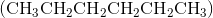to form liquid water and carbon dioxide gas.

 Type of Bond Average bond enthalpy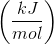Carbon-hydrogen single bond 414 Carbon-oxygen double bond 736 Oxygen-oxygen double bond 498 Oxygen-hydrogen single bond 464 Carbon-carbon single bond 347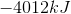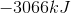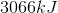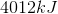Explanation:

Start by writing the balanced equation for the combustion of hexane: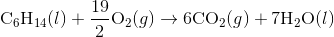Recall the following equation: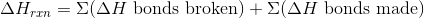Remember that energy is required to break bonds, so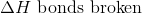should be positive. Energy is also released when bonds are made so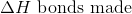should be negative.

Next, draw out the Lewis structures of each molecule to figure out the number of bonds made or broken.

For the hexane, there are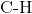bonds that must be broken, and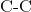bonds that must be broken.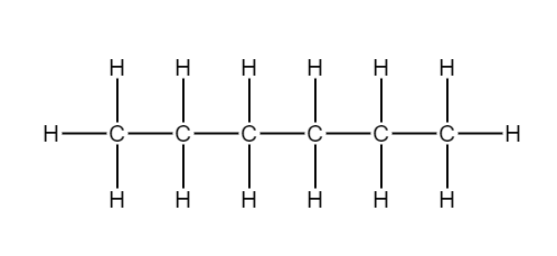For each oxygen, there is only 1 oxygen double bond to break. However, we will need to multiply this number by its stoichiometric coefficient.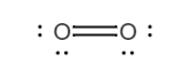For each carbon dioxide, there aredouble bonds between carbon and oxygen to break. For the given equation, there aretotal of these double bonds because we havemoles of carbon dioxide.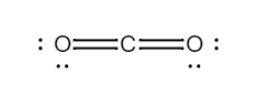For each water, there aresingle bonds between hydrogen and oxygen. For the given equation, there are a total ofof these bonds because we havemoles of water.

Now, use the given information regarding to average bond enthalpies to find the change in enthalpy for the reaction.